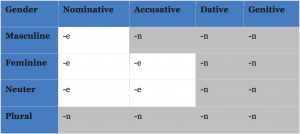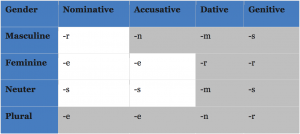# Simplifying the German Declension (Part II)

After my first post about the declension of the determiners, now I’m going to explain how the declension of the adjectives works in German.

1. In general, it is different from the ending of the determiner preceding the adjective (only -e and -n can coincide).
2. It depends on what determiner is used, or even if there is none.

### 1. The endings of the adjective

In most cases, the adjective has only to different endings: -e or -n.(Notice: Like in the case of the determiner, the -n includes automatically an “e”: großen.)

• “grey” corresponds to -n.

### 2. How the declension depends on the determiner

There are 3 possibilities of determiner endings:

1. Declined like the definite article: dieser, jener, welcher.
2. Declined like the indefinite article: kein, mein, dein, etc.
3. There is no determiner.

In the first case, you have to apply the table as you see it above.

Examples:

• Nominative neuter: Das ist das neue Auto.
• Accusative masculine: Ich habe einen großen Hund.

In the second case, there are two exceptions from the table above. These exceptions are:

• Nominative masculine: the adjective ends in -r.
• Nominative and accusative neuter: -s (these are exactly the two letters you learned in my first post about the German declension).

Examples:

• Nominative masculine: Das ein netter Lehrer (instead of nette).
• Accusative neuter: Ich sehe dein neues Auto (instead of neue).

In the third case (no determiner used), you have to use the endings of the table in my first post about the German declension (the so-called “letters”).

Examples:

• Nominative plural: Da sind viele Leute (letter “-e” for plural, not the adjective ending with determiner “-en”!).
• Dative feminine: Kaffee mit heißer Milch.

### 3. Merging both declensions in one

Now, I’m going to make a synthesis of both tables: the table of my first post and the table of this post together. How can that be possible, different endings for the determiner and the adjective in just one table?

Easy: The first word (determiner) takes as ending the letter of my first table (compare the letters↓, they are exactly the same as in my first post).

And the second word (adjective) takes as ending the letter that corresponds to one of the to (shading) colors: “white” (or “no shading”) = -e, “grey (shading)” = -n.Examples:

• Nominative masculine: De-r (letter) neu-e (white color) Lehrer kommt zum Unterricht.
• Dative feminine: Helge kommt mit seine-r (letter) neue-n (grey color) Freundin.